Search IntMath
Close

# Infinite series background

By Murray Bourne, 28 Jun 2018

I recently developed interactive applets that demonstrate how Taylor and Maclaurin Series and the Binomial Series approximate a given curve.

I wondered about the story behind infinite series. Why were they developed? Who developed them? What problems were they trying to solve?

## Infinite series

I'm standing 5 m from a wall. I jump half the distance (2.5 m) towards the wall. I halve the distance again (1.25 m) and continue getting closer to the wall by stepping half the remaining distance each time. Do I ever reach the wall?

Zeno, the 5th century BCE Greek philosopher, proposed a similar question in his famous Paradoxes.

One of the best known infinite series is the following, related to Zeno's Paradox: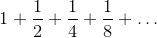If you keep adding smaller and smaller fractions following this pattern, you'll find your answer gets closer and closer to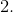When this happens, we say the infinite series converges to a value (but in many cases series will diverge - just keep getting bigger and bigger).

## Archimedes' parabola segment

The first known example of an infinite sum was when Greek mathematician Archimedes showed in the 3rd century BCE that the area of a segment of a parabola is 4/3 the area of a triangle with the same base. The notation he used was different, of course, and some of the approach was more geometric than algebraic, but his approach of summing infinitely small quantities was quite remarkable for the time.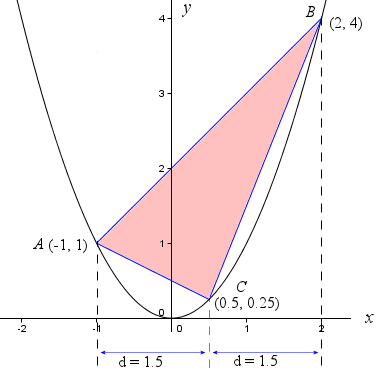See the details here:

## The motivation

Throughout history, mathematicians tried to find reliable ways to calculate the area and volume of objects that didn't have straight or regular sides, and to precisely describe non-constant motion (displacement, velocity, acceleration).Antique clock, c. 1800

Another important issue faced by scientists of the 17th and 18th centuries was to improve navigation of ships at sea. Captains needed to know exactly where they were on the Earth, and better clocks, better understanding of longitude and latitude, and the movement of stars and planets were all achieved through studies of motion and the development of calculus.

## Newton's binomial series

One of Isaac Newton's achievements was the development of the binomial series, which allows us to express the power, n (rational) of a binomial (2-term) expression as an infinte series: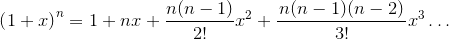This gave a useful way to approximate the value of various functions, but more importantly, it led to the development of calculus. Here are some examples: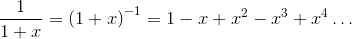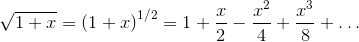Such power series, along with calculus, were major advances in mathematics and science at the turn of the 18th century.

The approach enabled term-by-term integration. For example, in the two cases above, if we don't know how to integrate the left hand side, we can easily integrate each term on the right hand side to obtain a good approximation.

Power series also helped establish sine, cosine, log, etc as "functions".

Most importantly, for Newton (and his contemporaries, like Leibniz) power series were inextricably linked with calculus.

See:

## Taylor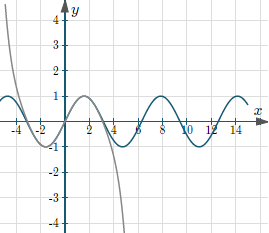Approximation of f(x) = sin(x) by Taylor series

While Indian and Chinese mathematicians developed various infinite expansions for some trigonometric functions, Brook Taylor developed a general method for writing a broad range of functions as infinite series in 1715. The Taylor Series involves knowing the derivative of the function, and the expansion around some value x = a is as follows: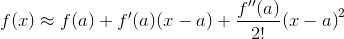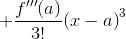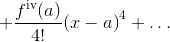See examples at: Taylor Series

## Maclaurin

Scottish mathematician Colin Maclaurin (who began university at age 11), in A Treatise of Fluxions published in 1742, explored a special case of Taylor's series, expanded around x = 0. This is now known as Maclaurin's Series.

See examples at: Maclaurin Series

## Euler

In Leonhard Euler's Introductio in analysin infinitorum (Introduction to Analysis of the Infinite, 1748), we see further development of power series into the definition of a function.

Euler derived series for sine, cosine, exp, log, etc., and he also discovered relationships between them, for example: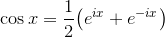Euler also introduced sigma notation (Σ) for sums of series. So we could write (e.g.)more concisely as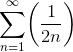For an English translation, see: Introductio in analysin infinitorum

## Lagrange

Joseph-Loius Lagrange, in his Theorie des fonctions analytiques (1797) attempted to liberate calculus from the notion of infinitely small quantities, the basis of Newton and Lebniz' ideas. Lagrange worked on solving differential equations, a branch of calculus.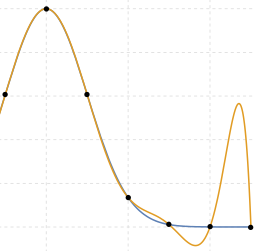Lagrange polynomial [source]

He developed a new approach to interpolation and Taylor series, where the aim is to find a polynomial passing through a given set of points.

Be the first to comment below.

### Comment Preview

HTML: You can use simple tags like <b>, <a href="...">, etc.

To enter math, you can can either:

1. Use simple calculator-like input in the following format (surround your math in backticks, or qq on tablet or phone):
a^2 = sqrt(b^2 + c^2)
(See more on ASCIIMath syntax); or
2. Use simple LaTeX in the following format. Surround your math with $$ and $$.
$$\int g dx = \sqrt{\frac{a}{b}}$$
(This is standard simple LaTeX.)

NOTE: You can mix both types of math entry in your comment.